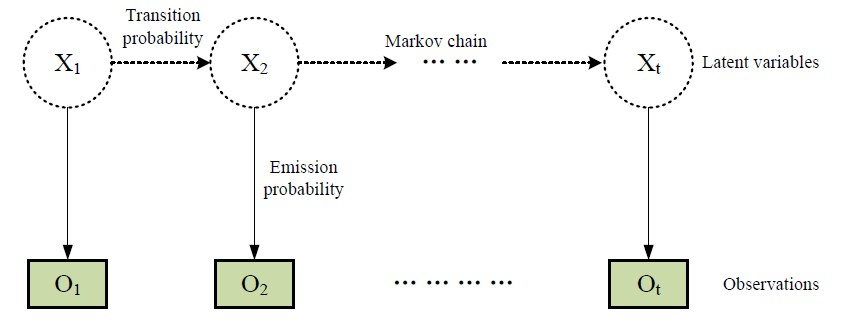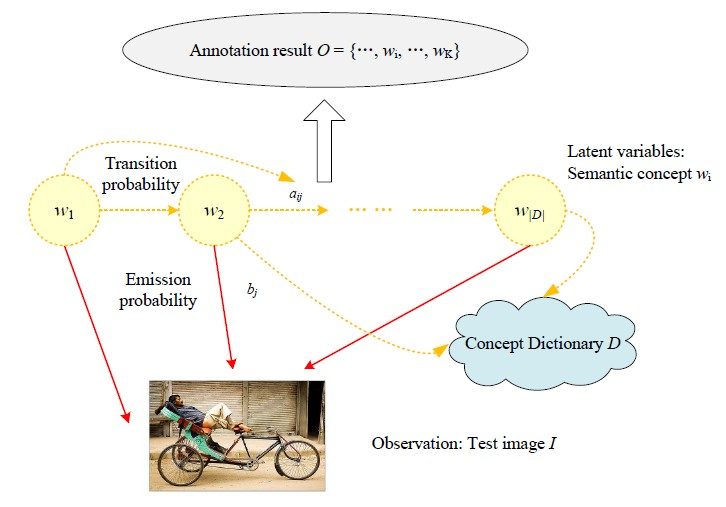﻿ 基于隐马尔科夫模型和卷积神经网络的图像标注方法 Automatic Image Annotation Based on Hidden Markov Model and Convolutional Neural Network

Computer Science and Application
Vol.08 No.09(2018), Article ID:26629,8 pages
10.12677/CSA.2018.89141

Automatic Image Annotation Based on Hidden Markov Model and Convolutional Neural Network

Haijiao Xu, Qionghao Huang, Fan Wang, Yao Wen, Meihua Zhao

School of Information Technology in Education, South China Normal University, Guangzhou GuangdongReceived: Aug. 6th, 2018; accepted: Aug. 21st, 2018; published: Aug. 28th, 2018ABSTRACT

Automatic image annotation is becoming increasingly important in order to develop algorithms that are able to search and browse large-scale image databases. In this paper, we propose a novel annotation approach termed HMM + CNN, which is based on Hidden Markov Model (HMM) and Convolutional Neural Network (CNN). First, a multi-label CNN is trained as a concept classifier. Then, through a first-order HMM, image content and semantics correlation is combined to refine the predicted semantic scores. Finally, to improve the performance of labeling rare concepts, the gradient descent algorithm is applied for compensating the varying frequencies of concepts derived from imbalanced image datasets. Experiments have been carried out on IAPR TC-12 image annotation database. The results show that our proposed approach performs favorably compared with several conventional methods.

Keywords:Automatic Image Annotation, Hidden Markov Model, Convolutional Neural Network, Multi-Label Learning1. 引言

2. 隐马尔科夫模型

3. 基于HMM与CNN的自动图像标注方法

3.1. 问题描述Figure 1. The graphical illustration of hidden Markov model

3.2. 发射概率估算

CNN分类器产生的视觉相关性分数可视为相应隐状态的发射概率bj，该发射概率表示了CNN分类器把图像I映射到语义概念wj的过程。任何CNN网络都可融入我们的标注模型，不失一般性，我们选择了近年来一个有影响力的高效CNN模型ResNet  来作为我们的CNN分类器。Figure 2. The HMM + CNN framework

$p\left({w}_{j}|I\right)=\frac{\mathrm{exp}\left({q}_{j}\left(I\right)\right)}{{\sum }_{k}\mathrm{exp}\left({q}_{k}\left(I\right)\right)}$ , (1)

${f}_{\text{softmax}}=-\frac{1}{N}{\sum }_{i}{\sum }_{j}{\overline{p}}_{i,j}\mathrm{log}\left(p\left({w}_{j}|I\right)\right)$ , (2)

3.3. 转移概率估算

${a}_{ij}=\frac{|S\left({w}_{i}\right)\cap S\left({w}_{j}\right)|}{|S\left({w}_{i}\right)|}$ (3)

3.4. HMM + CNN图像标注算法

1 构建状态转移矩阵A = {aij|i,j = 1, ∙∙∙, N};

2 构建发射概率矩阵B = {bj|j = 1, ∙∙∙, N};

3 初始化标注结果集 ${O}_{1}={\mathrm{max}}_{1\le j\le N}\left({b}_{j}\right)\text{,}\text{\hspace{0.17em}}\text{O}={O}_{1}$

4 for k = 2 to K do

5 设上一步检索概念Ok−1 = wi，则 ${O}_{j}={\mathrm{max}}_{1\le j\le N}\left({p}_{1}{b}_{j}+{p}_{2}{a}_{ij}\right)$

6 $O=O\cup {O}_{j}$

7 end for

8 返回结果集O。

4. 实验和评价

4.1. 数据集

4.2. 实验结果与分析

1) 初始化权重向量，即P1 = 0, P2 = 1 − P1；

2) 对于任意的i(1 ≤ I ≤ N)：

2a) 对于不同的权重p1 Î {0, 0.1, ∙∙∙, 1}执行算法1获得标注结果集O并记录最大F1性能分数，写入相对应的权重值pmi：P1i = pmi, P2i = 1 − P1iTable 2. Performance comparisons of multi-label image annotation

2b) i值加一，即i = i + 1；

3) 输出权重向量P1, P2。

Automatic Image Annotation Based on Hidden Markov Model and Convolutional Neural Network[J]. 计算机科学与应用, 2018, 08(09): 1309-1316. https://doi.org/10.12677/CSA.2018.89141

1. 1. Chang, C.C. and Lin, C.J. (2011) LIBSVM: A Library for Support Vector Machines. ACM Transactions on Intelligent Systems and Technology, 2, 1-27. https://doi.org/10.1145/1961189.1961199

2. 2. Ballan, L., Uricchio, T., Seidenari, L. and Bimbo, A.D. (2014) A Cross-Media Model for Automatic Image Annotation. International Conference on Multimedia Retrieval, 73. https://doi.org/10.1145/2578726.2578728

3. 3. Moran, S. and Lavrenko, V. (2014) Sparse Kernel Learning for Image Annotation. International Conference on Multimedia Retrieval, 113-120. https://doi.org/10.1145/2578726.2578734

4. 4. Chen, M., Zheng, A. and Weinberger, K. (2013) Fast Image Tagging. International Conference on Machine Learning, 1274-1282.

5. 5. Murthy, V.N., Can, E.F. and Manmatha, R. (2014) A Hybrid Model for Automatic Image Annotation. International Conference on Multimedia Retrieval, 369-376. https://doi.org/10.1145/2578726.2578774

6. 6. Jin, C. and Jin, S.W. (2016) Image Distance Metric Learning Based on Neighborhood Sets for Automatic Image Annotation. Journal of Visual Communication and Image Representation, 34, 167-175. https://doi.org/10.1016/j.jvcir.2015.10.017

7. 7. Ji, P., Gao, X. and Hu, X. (2017) Automatic Image Annotation By Combining Generative and Discriminant Models. Neurocomputing, 236, 48-55. https://doi.org/10.1016/j.neucom.2016.09.108

8. 8. 周铭柯, 柯逍, 杜明智. 基于数据均衡的增进式深度自动图像标注[J]. 软件学报, 2017, 28(7): 1862-1880.

9. 9. Ma, Y., Liu, Y., Xie, Q. and Li, L. (2018) CNN-Feature Based Automatic Image Annotation Method. Multimedia Tools & Applications, 1-14. https://doi.org/10.1007/s11042-018-6038-x

10. 10. Mojoo, J., Kurosawa, K. and Kurita, T. (2017) Deep CNN with Graph Laplacian Regularization for Multi-Label Image Annotation. International Conference on Image Analysis and Recognition, 19-26. https://doi.org/10.1007/978-3-319-59876-5_3

11. 11. Grubinger, M., Clough, P. and Müller, H. (2006) The IAPR Benchmark : A New Evaluation Resource for Visual Information Systems. International Conference on Language Resources and Evaluation, 13-23.

12. 12. Saini, R., Roy, P. and Dogra, D. (2018) A Segmental HMM Based Trajectory Classification Using Genetic Algorithm. Expert System Application, 93, 169-181. https://doi.org/10.1016/j.eswa.2017.10.021

13. 13. He, K., Zhang, X., Ren, S. and Sun, J. (2016) Deep Residual Learning for Image Recognition. IEEE Conference on Computer Vision and Pattern Recognition, 770-778.